# NMath User's Guide

17.5 Banded Matrices (.NET, C#, CSharp, VB, Visual Basic, F#)

A banded matrix is a matrix that has all its non-zero entries near the diagonal. Entries farther above the diagonal than the upper bandwidth, or farther below the diagonal than the lower bandwidth, are defined to be zero. That is, ifis the upper bandwidth, and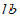is the lower bandwidth, then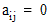whenever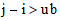or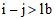.

For example, this is a 7 x 7 banded matrix with upper bandwidth 1 and lower bandwidth 3: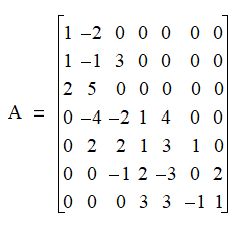NMath provides banded matrix classes for four datatypes: single- and double-precision floating point numbers, and single- and double-precision complex numbers. The classnames are , , , and .

For efficiency, zero elements outside the bandwidth are not stored. Instead, matrix values are stored in a vector column by column. Blank entries are inserted in the data vector so that the each column takes up the same number of elements,, in the vector. For example, the following 8 x 8 matrix with an upper bandwidth of 2 and a lower bandwidth of 1: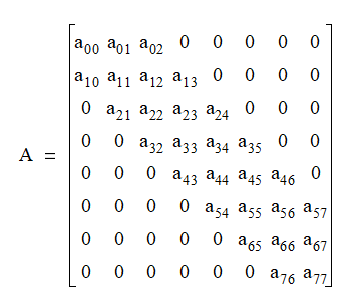is stored in a data vector as:

```v = [x   x   a00 a10
```
```     x   a01 a11 a21
```
```     a02 a12 a22 a32
```
```     a13 a23 a33 a43
```
```     a24 a34 a44 a54
```
```     a35 a45 a55 a65
```
```     a46 a56 a66 a76
```
```     a57 a67 a77 x  ]
```

where x denotes an unused location.

Top

Top